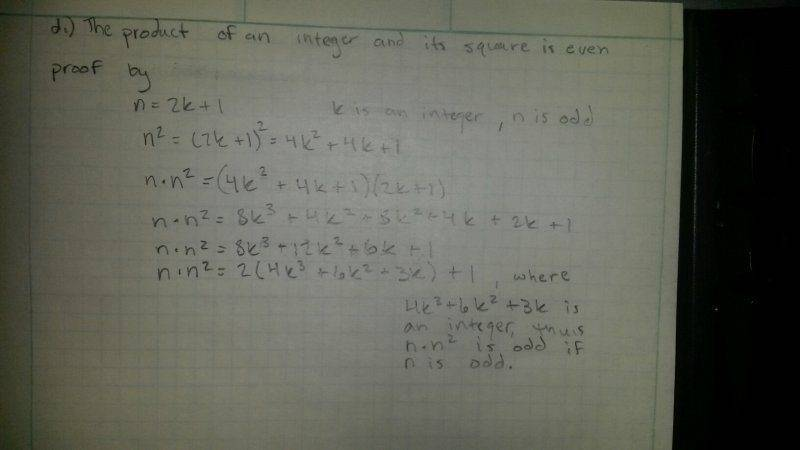# What type of proof is this defined as?

## Homework Statement

I have to prove or disprove the following statement.

The product of an integer and its square is even

## Homework Equations

n2*n
n = 2k + 1 (Odd integers)[/B]

## The Attempt at a SolutionI uploaded a photo of my proof. As you can see, I have proved that if n is an odd integer, the product of n squared and n will be odd as well. My question is what type of proof is this? I was thinking it was proof by counterexample but I wasn't sure if the correct terminology would be "disproof by counterexample". Or is it a proof by contradiction? Any help is appretiated : )

Mark44
Mentor

## Homework Statement

I have to prove or disprove the following statement.

The product of an integer and its square is even

## Homework Equations

n2*n
n = 2k + 1 (Odd integers)[/B]

## The Attempt at a Solution

View attachment 73597
I uploaded a photo of my proof. As you can see, I have proved that if n is an odd integer, the product of n squared and n will be odd as well. My question is what type of proof is this? I was thinking it was proof by counterexample but I wasn't sure if the correct terminology would be "disproof by counterexample". Or is it a proof by contradiction? Any help is appretiated : )
This is a direct proof. You proved the given statement, so what you did is not a disproof. You can't prove a statement by counterexample. To prove an implication by contradiction, you assume the opposite of the conclusion of the implication, and then show that this causes a contradiction.

BTW, where you show several lines that start with "n * n2 = ", you could just continue the line above using '=' and omit the "n * n2 = " part.

•Epond89
Fredrik
Staff Emeritus
Science Advisor
Gold Member
The problem is asking about the claim "the product of an integer and its square is even". This claim should be interpreted as "For all integers n, ##n n^2## is even". To prove that this statement is false, you only need to find one counterexample, i.e. one integer n such that ##nn^2## is odd.

What you actually did was to prove the statement "For all odd integers n, ##nn^2## is odd". This is a theorem that you can use to prove that the given statement is false, but you didn't actually say how your theorem implies that the given statement is false, so technically, you haven't completed the proof. The final step is pretty trivial though. All you have to do is to say that your theorem implies that every odd integer is a counterexample that proves the given claim false. You can also be more specific, and say e.g. that your theorem proves that 1 is a counterexample that proves the given claim false. (But you wouldn't actually need your theorem to see that).

•Epond89